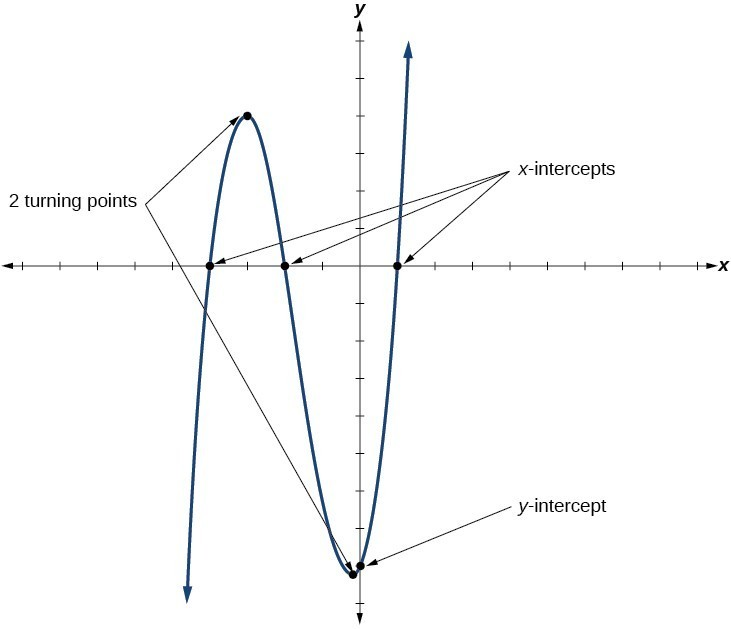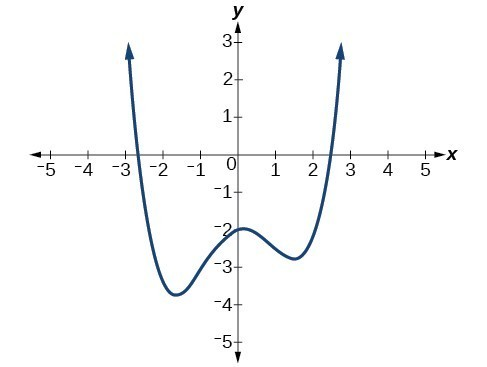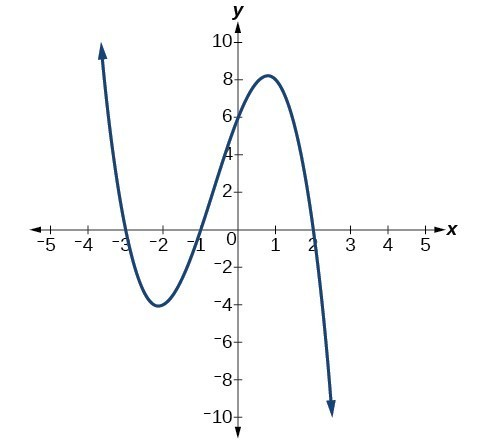## Local Behavior of Polynomial Functions

### Learning Outcomes

• Identify turning points of a polynomial function from its graph.
• Identify the number of turning points and intercepts of a polynomial function from its degree.
• Determine x and y-intercepts of a polynomial function given its equation in factored form.

## Identifying Local Behavior of Polynomial Functions

In addition to the end behavior of polynomial functions, we are also interested in what happens in the “middle” of the function. In particular, we are interested in locations where graph behavior changes. A turning point is a point at which the function values change from increasing to decreasing or decreasing to increasing.We are also interested in the intercepts. As with all functions, the y-intercept is the point at which the graph intersects the vertical axis. The point corresponds to the coordinate pair in which the input value is zero. Because a polynomial is a function, only one output value corresponds to each input value so there can be only one y-intercept $\left(0,{a}_{0}\right)$. The x-intercepts occur at the input values that correspond to an output value of zero. It is possible to have more than one x-intercept.

### A General Note: Intercepts and Turning Points of Polynomial Functions

• A turning point of a graph is a point where the graph changes from increasing to decreasing or decreasing to increasing.
• The y-intercept is the point where the function has an input value of zero.
• The x-intercepts are the points where the output value is zero.
• A polynomial of degree n will have, at most, n x-intercepts and n – 1 turning points.

## Determining the Number of Turning Points and Intercepts from the Degree of the Polynomial

A continuous function has no breaks in its graph: the graph can be drawn without lifting the pen from the paper. A smooth curve is a graph that has no sharp corners. The turning points of a smooth graph must always occur at rounded curves. The graphs of polynomial functions are both continuous and smooth.

The degree of a polynomial function helps us to determine the number of x-intercepts and the number of turning points. A polynomial function of nth degree is the product of n factors, so it will have at most n roots or zeros, or x-intercepts. The graph of the polynomial function of degree n must have at most n – 1 turning points. This means the graph has at most one fewer turning point than the degree of the polynomial or one fewer than the number of factors.

### Example: Determining the Number of Intercepts and Turning Points of a Polynomial

Without graphing the function, determine the local behavior of the function by finding the maximum number of x-intercepts and turning points for $f\left(x\right)=-3{x}^{10}+4{x}^{7}-{x}^{4}+2{x}^{3}$.

The following video gives a 5 minute lesson on how to determine the number of intercepts and turning points of a polynomial function given its degree.

### Try It

Without graphing the function, determine the maximum number of x-intercepts and turning points for $f\left(x\right)=108 - 13{x}^{9}-8{x}^{4}+14{x}^{12}+2{x}^{3}$

### How To: Given a polynomial function, determine the intercepts

1. Determine the y-intercept by setting $x=0$ and finding the corresponding output value.
2. Determine the x-intercepts by setting the function equal to zero and solving for the input values.

## Using the Principle of Zero Products to Find the Roots of a Polynomial in Factored Form

The Principle of Zero Products states that if the product of n numbers is 0, then at least one of the factors is 0. If $ab=0$, then either $a=0$ or $b=0$, or both a and b are 0. We will use this idea to find the zeros of a polynomial that is either in factored form or can be written in factored form. For example, the polynomial

$P(x)=(x-4)^2(x+1)(x-7)$

is in factored form. In the following examples, we will show the process of factoring a polynomial and calculating its x and y-intercepts.

### Example: Determining the Intercepts of a Polynomial Function

Given the polynomial function $f\left(x\right)=\left(x - 2\right)\left(x+1\right)\left(x - 4\right)$, written in factored form for your convenience, determine the y and x-intercepts.

### Example: Determining the Intercepts of a Polynomial Function BY Factoring

Given the polynomial function $f\left(x\right)={x}^{4}-4{x}^{2}-45$, determine the y and x-intercepts.

### Try It

Given the polynomial function $f\left(x\right)=2{x}^{3}-6{x}^{2}-20x$, determine the and x-intercepts.

## The Whole Picture

Now we can bring the two concepts of turning points and intercepts together to get a general picture of the behavior of polynomial functions.  These types of analyses on polynomials developed before the advent of mass computing as a way to quickly understand the general behavior of a polynomial function. We now have a quick way, with computers, to graph and calculate important characteristics of polynomials that once took a lot of algebra.

In the first example, we will determine the least degree of a polynomial based on the number of turning points and intercepts.

### Example: Drawing Conclusions about a Polynomial Function from Its Graph

Given the graph of the polynomial function below, determine the least possible degree of the polynomial and whether it is even or odd. Use end behavior, the number of intercepts, and the number of turning points to help you.Now you try to determine the least possible degree of a polynomial given its graph.

### Try It

Given the graph of the polynomial function below, determine the least possible degree of the polynomial and whether it is even or odd. Use end behavior, the number of intercepts, and the number of turning points to help you.Now we will show that you can also determine the least possible degree and number of turning points of a polynomial function given in factored form.

### Example: Drawing Conclusions about a Polynomial Function from ITS Factors

Given the function $f\left(x\right)=-4x\left(x+3\right)\left(x - 4\right)$, determine the local behavior.

Now it is your turn to determine the local behavior of a polynomial given in factored form.

### Try It

Given the function $f\left(x\right)=0.2\left(x - 2\right)\left(x+1\right)\left(x - 5\right)$, determine the local behavior.

## Contribute!

Did you have an idea for improving this content? We’d love your input.